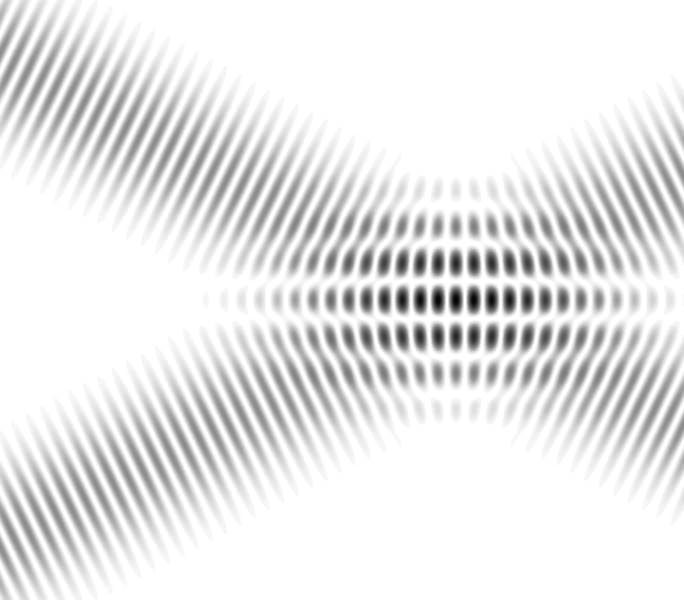Interference (wave propagation) – Wikipedia, the free encyclopediaThe principle of superposition of waves states that when two or more propagating waves of same type are incident on the same point, the total displacement at that point is equal to the pointwise sum of the displacements of the individual waves. If a crest of a wave meets a crest of another wave of the same frequency at the same point, then the magnitude of the displacement is the sum of the individual magnitudes – this is constructive interference. If a crest of one wave meets a trough of another wave then the magnitude of the displacements is equal to the difference in the individual magnitudes – this is known as destructive interference.